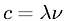Chemistry > Atomic StructureShowing results 1 to 8 of 8, on page 1 of 1
 DESCRIPTION EQUATION Bohr RadiusDe Broglie Wavelength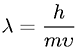Linear Momentum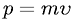Planck's Quantized (Quantum) Energy Equation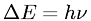Radii of stable orbits in the Bohr model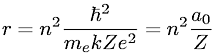Relationship between Energy and Principal Quantum Number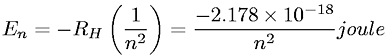Rydberg EquationSpeed of Light to Wavelength and Frequency Relationship